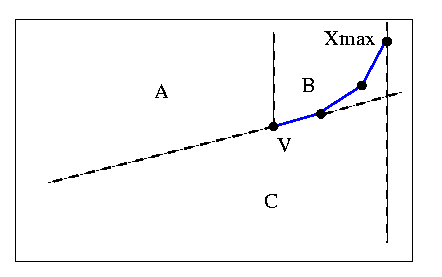Ghosh & Shyamasundar 1983

Ghosh and Shyamasundar presented an algorithm  which seems to be quite simple as an idea. They begin with X-max and proceed towards X-min, using monotonicity to compute the lower hull. Then they repeat similarly for the upper hull. The authors claim that Lee's algorithm is "not simple either from the algorithmic point of view or from the proof of validity point of view". Personally I found Lee's paper to be one of the easiest to read. Furthermore it turns out that their own algorithm was too simple, since Toussaint and Shin and Woo found counterexamples (private communication between the authors). There are also several minor "bugs" in their algorithm. The authors presented a revised edition later on, which is in no way as simple as Lee's algorithm.

I will illustrate the main steps, apart from small details like collinearities and the initial/final steps.

Imagine we have a developing lower convex chain starting from Xmax, as shown below. The vertices are maintained in a stack. The top element is V. The regions where the next vertex of the polygon can be are labeled A,B,C, as shown.If the next vertex is in (A), it is pushed onto the stack.

If the next vertex is in (B), monotonicity in the horizontal direction is violated. The vertex is ignored.

If the next vertex is in (C), V is deleted since it is concave, and the algorithm backtracks.

In the above figure, if the next vertex is in (A), it can be added since the chain will remain convex.

If the next vertex is in (B), the algorithm ignores it and all following vertices that remain in B. Finally a vertex must emerge through the vertical half-line above V. This vertex will be in region (A) or (C).

If the next vertex is in (C), the algorithm backtracks, deleting all concave vertices.

These simple steps seem to be reasonable, when applied individually to the figure above. The problem with the logic of the algorithm is that this figure is not maintained after every iteration. The best way to illustrate this is through the following counterexample, which is similar to the counterexample that Toussaint provided to Sklanskys' revised algorithm.When the stack contains Xmax, 2, 3, and vertex 4 is next to be examined, it is in region (C), so (3) is deleted. The algorithm backtracks, so (2) is the top of the stack, and (4) is in region (A). Therefore (4) is pushed onto the stack which now contains Xmax,2,4. According to the algorithm, the next vertex (5) is now in region (A), since (4) is the top of the stack. Then (6) is in region (B) when (5) is the top of the stack. Notice that edge 5-6 crosses the convex chain of the stack. Since (6) is in (B), it is deleted. As you can see, (6) should be on the convex hull.

The algorithm assumes that once the chain enters region (B), it must exit as was originally described. This is obvisouly wrong. Finally I present three more errors that I have found:

According to the notation that the authors use, execution of the algorithm is aborted when the next vertex is exactly on the half-line above V, since they do not have a case to handle this situation.

If the first vertex after Xmax is Xmin, then Xmin is pushed onto the stack twice.

If there is one vertex between Xmax and Xmin, and this vertex is concave, the algorithm deletes it, but then proceeds into the upper hull without even realizing that it has done so. It then proceeds to delete all points except Xmax and then enters into an infinite loop.

previous : ElGindy, Avis and Toussaint 1983.          next : Bhattacharya and ElGindy 1984.